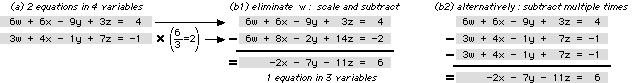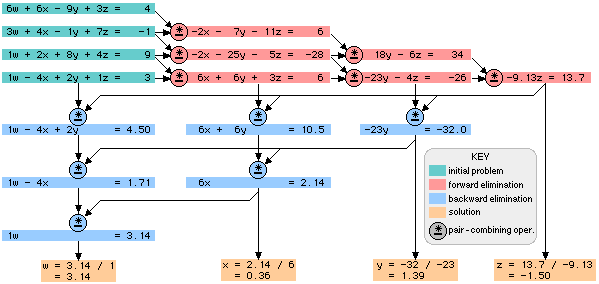ABC An ABC Operator's Manual:1. Principles of the Solution Process

The solution process of the ABC is based on the Gaussian elimination method for solving a set of linear equations. The basic principle underlying Gaussian elimination is that 2 equations in N variables can be combined to produce 1 equation in N-1 variables. For linear equations, this can be accomplished by scaling one of the equations and subtracting it from the other. The scale factor is the ratio of the coefficients of the variable to be eliminated. See figure 1.1a and 1.1b1.

Figure 1.1: Pair-Combining OperationThe division and multiplications of the scaling can be avoided by performing multiple subtractions or additions, along with shifting, in a manner similar to common division algorithms, as shown in figure 1.1b2. The ABC uses this scheme to implement the pair-combining operation.

To solve a set of N equations in N variables (green in figure 1.2), this pair-combining operation is performed successively (red) until 1 equation in 1 variable remains, thus providing the solution for one of the variables. Following this, another succession of pair-combining operations (blue) results in 1 equation in 1 variable for each of the remaining variables. These two successions of operations are referred to as forward elimination and backward elimination. The resultant set of N equations in 1 variable are then solved to yield the values of the variables (orange).

Figure 1.2: Forward and Backward Elimination (N=4)There is latitude in the choice of equations which enter the backward elimination stage: within a given column, any of the green or red equations may be selected for entry into the backward elimination stage. Further, in a given column during backward elimination, the order of eliminations is flexible.

When using the ABC, the machine will perform the pair-combining operation automatically while the human operator is responsible for sequencing these operations through the forward and backward elimination process.

 1. Principles | 2. Stages | 3. Equipment | 4. Flowchart Proof-of-Concept | Architecture | ASM | Manual | Simulation ABC bhilpert2002